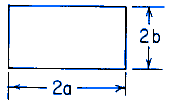Related Resources: calculators

### Solid Rectangular Section Torsional Deformation and Stress Torsional Deformation Stress Equation and Calculator

Beam Deflection and Stress Equation and Calculators

Torsional Deformation and Stress Solid Rectangular Section Equations and CalculatorALL calculators require a Premium Membership

θ = T L / K Gat the midpoint of each longer side for a ≥ b

Where:

θ - angle of twist (radians)
T = Twisting or torque moment force-length, (in-lbs, N-mm)
L = Length (in, mm)
a = Distance as indicated (in, mm)
b = Distance as indicated (in, mm)
τ = Unit shear stress force / area (lbs/in2, N/mm2)
G = Modulus of rigidity force / area (lbs/in2, N/mm2)
K = Polar Moment of Inertia (in4, mm4) for section

Reference:
Roarks Formulas for Stress and Strain, 7th Edition, Table 10.1 Formulas for torsional deformation and stress.